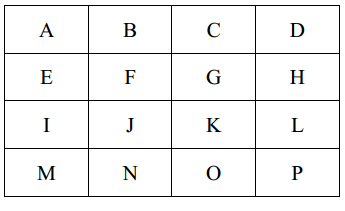2020-03-04 21:05

# c++基础问题，由于初学者，不会，求大神帮忙？• 点赞
• 写回答
• 关注问题
• 收藏
• 邀请回答

#### 1条回答默认 最新

•# 问题解决的话，请点下采纳

``````#include <iostream>
using namespace std;

int calc(char ch1, char ch2)
{
int n = 0;
int x1 = (ch1 - 'A') % 4;
int x2 = (ch2 - 'A') % 4;
int y1 = (ch1 - 'A') / 4;
int y2 = (ch2 - 'A') / 4;
if (x1 > x2) n += x1 - x2; else n += x2 - x1;
if (y1 > y2) n += y1 - y2; else n += y2 - y1;
return n;
}

int main()
{
char route;
cin >> route;
int sum = 0;
for (int i = 1; route[i] != 0; i++)
sum += calc(route[i], route[i - 1]);
cout << sum << endl;
return 0;
}
``````
点赞 评论